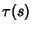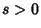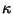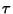## Fundamental Theorem of Space Curves

If two single-valued continuous functions(Curvature) and(Torsion) are given for, then there exists Exactly One Space Curve, determined except for orientation and position in space (i.e., up to a Euclidean Motion), whereis the Arc Length,is the Curvature, andis the Torsion.

See also Arc Length, Curvature, Euclidean Motion, Fundamental Theorem of Plane Curves, Torsion (Differential Geometry)

References

Gray, A. The Fundamental Theorem of Space Curves.'' §7.7 in Modern Differential Geometry of Curves and Surfaces. Boca Raton, FL: CRC Press, pp. 123 and 142-145, 1993.

Struik, D. J. Lectures on Classical Differential Geometry. New York: Dover, p. 29, 1988.